# Convolutional Neural Networks

CNN是深度学习中一种处理图像的神经网络，可以用来做图像的分类，segmentation，目标检测等等。其工作原理可简单概括为通过神经网络对图片特征的提取来达到我们想要的结果，相比于Full Connected网络，CNN需要学习的参数显著变少，相当于对图像进行了压缩。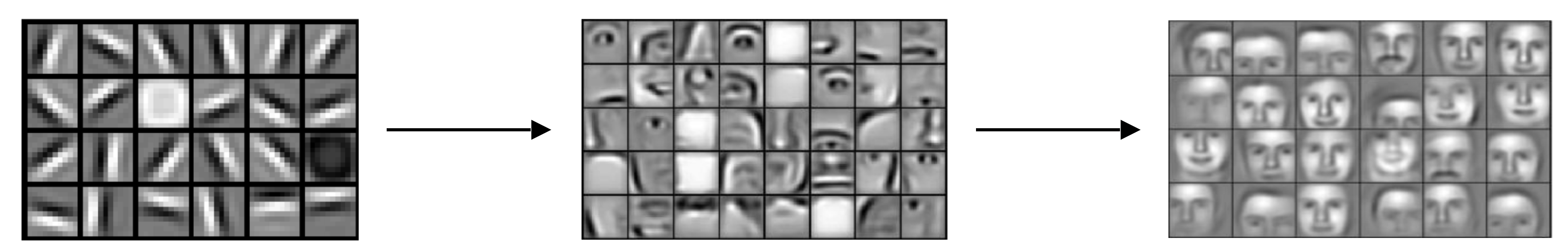### 卷积运算

CNN对图片特征的提取是基于卷积运算，由于图片中的像素点在空域上是离散的，因此准确的说是离散卷积运算。如果熟悉信号处理可知，卷积运算在频域上是一种滤波，比如图片的边缘包含大量的高频信息，我们可以设计一个高频滤波器对图片进行卷积，这样只有图片中的高频分量才能通过滤波器，即过滤出了图片的边缘。在空域上，卷积运算是一种加权求和的运算。其具体过程为让一个卷积核（卷积核）依次滑过图片中的所有区域，如下图所示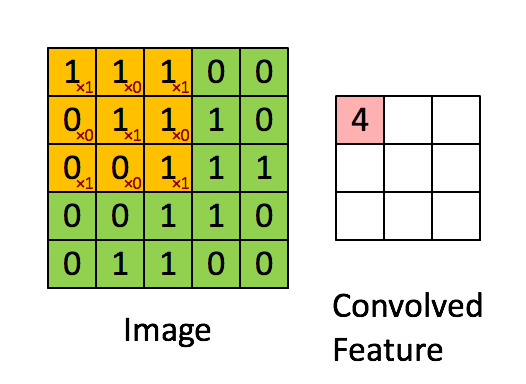### Strided Convolutions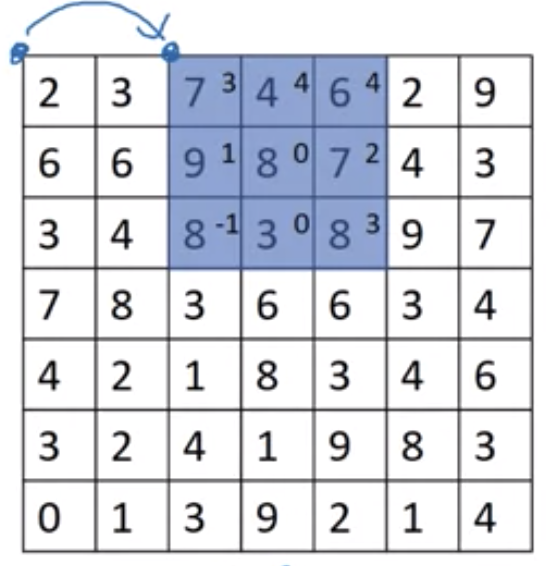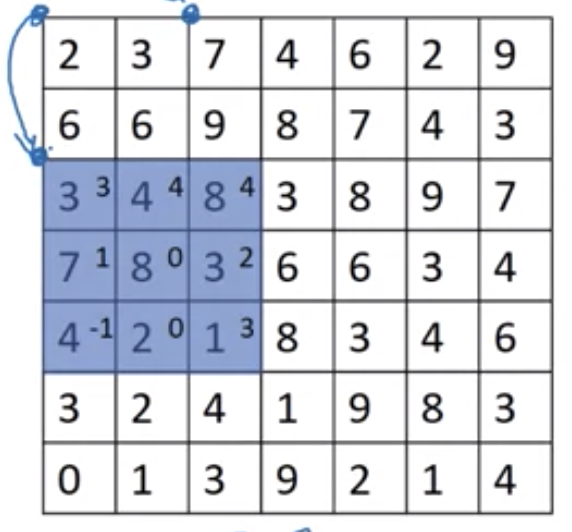### 三维卷积运算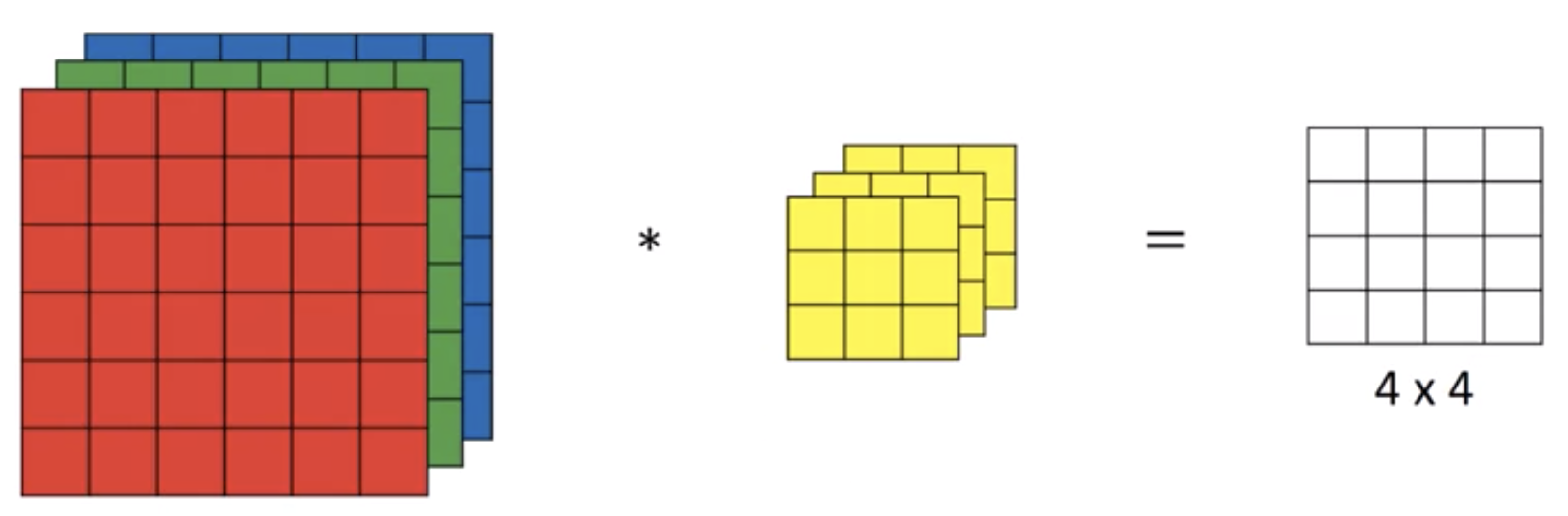r r r ... r
r r r ... r
... ... ...
r r r ... r
g g g ... g
g g g ... g
... ... ...
g g g ... g
b b b ... b
b b b ... b
... ... ...
b b b ... b


rgb rgb rgb ... rgb
rgb rgb rgb ... rgb
... ... ... ... rgb
rgb rgb rgb ... rgb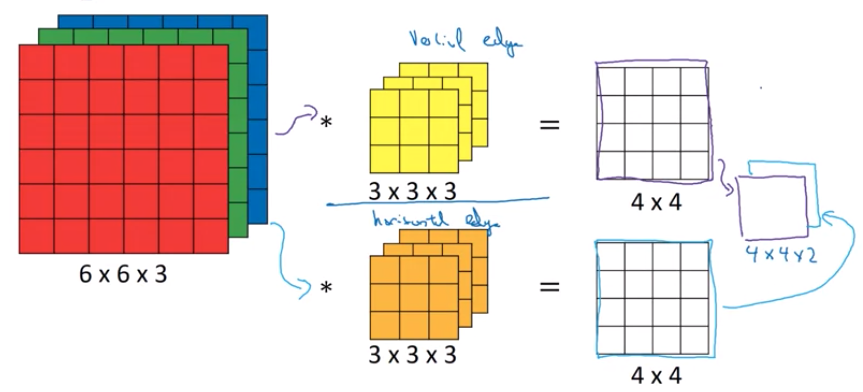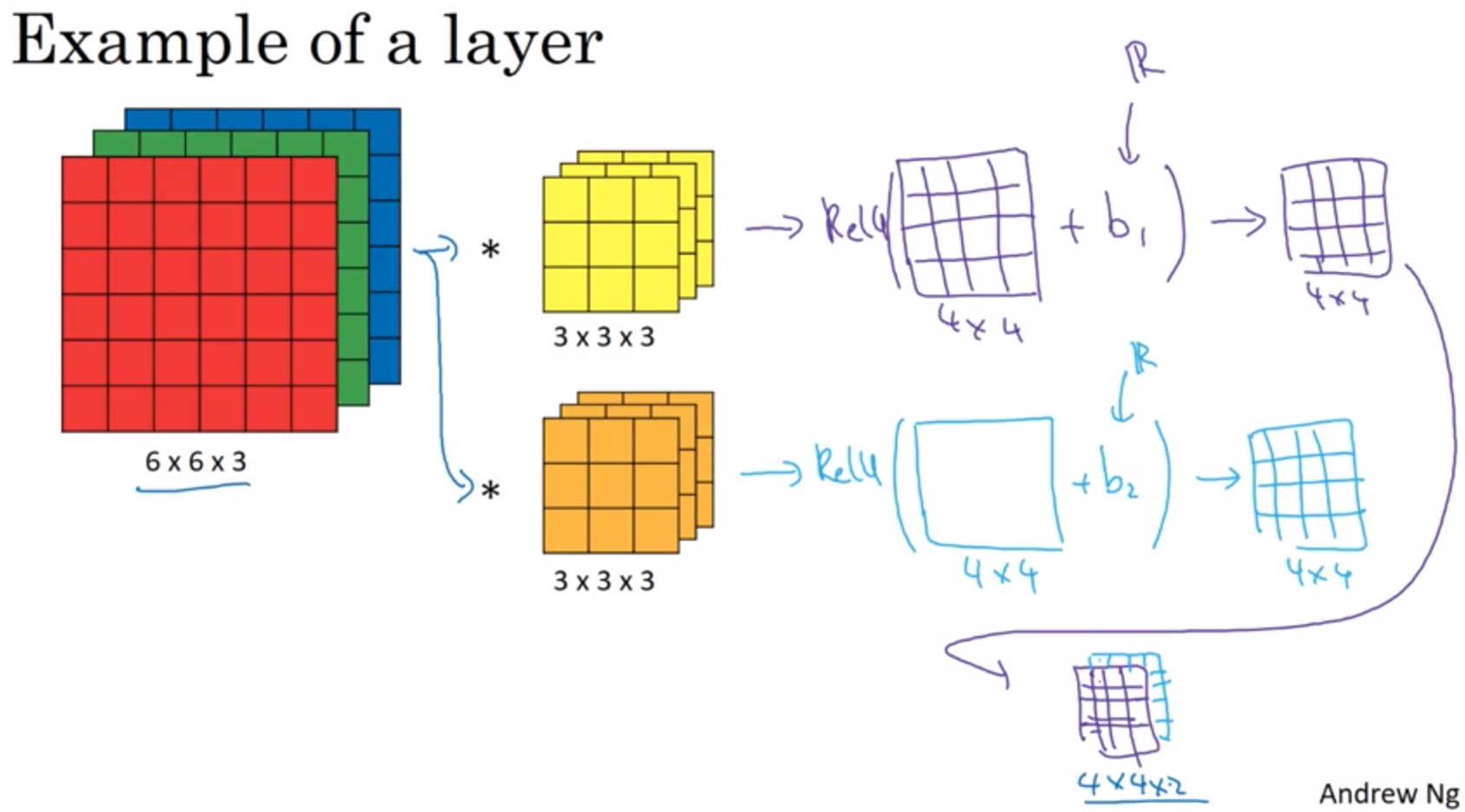• $f^{[l]}$ = filter size
• $p^{[l]}$ = padding
• $s^{[l]}$ = stride
• $n_C^{[l]}$ = number of filters

output的size为

Hidden Layer中每个Activation unit $A^{[l]}$的size为，其中m为batch数量

Weights $W^{[l]}$的size为

Bias $b^{[l]}$的size为 $n_C^{[l]}$

### Pooling

Pooling是用来对输入矩阵进行优化的一种方法。举例来说，下图是对一个4x4的矩阵进行max pooling，得到一个2x2的矩阵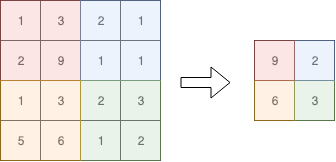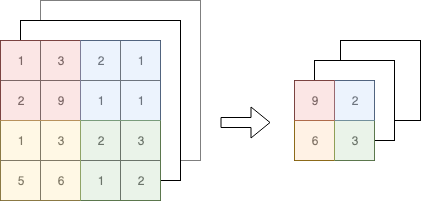### 一个完整的卷积神经网络

• Convolution
• Pooling
• Fully connected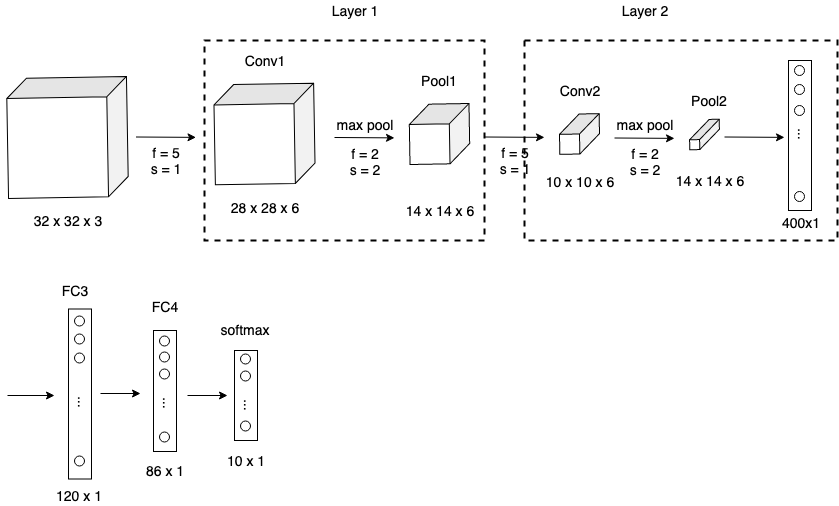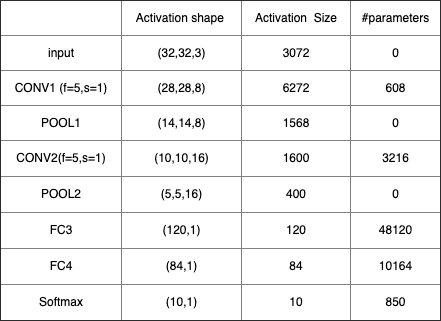• Parameter Sharing

• Sparsity of connections